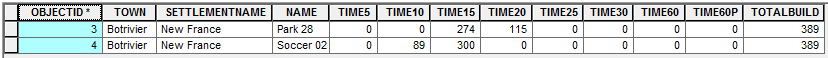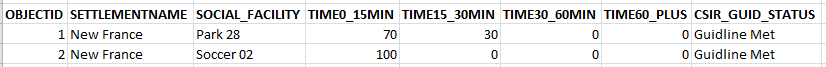# Data Access Module vs NumPy: Summarise Table

6879
8
05-17-2016 07:16 AMRegular Contributor

I'd like to find out what approaches the community have used to achieve similar results using either:

• Data Access Module or;
• NumPy

The following table (stats_table1) is my starting point:I'd like to populate a new table (stats_table2) based on the following structure:for each row in the first table:

• stats_table2 [SETTLEMENTNAME] = stats_table1 [SETTLEMENTNAME]
• stats_table2 [SOCIAL_FACILITY] = stats_table1 [NAME]
• stats_table2 [TIME0_15MIN] = stats_table1 (((TIME5 + TIME10 + TIME15)) / TOTALBUILD)*100)
• stats_table2 [TIME15 _30MIN] = stats_table1 (((TIME20 + TIME25 + TIME30)) / TOTALBUILD)*100)
• stats_table2 [TIME30 _60MIN] = stats_table1 ((TIME60 / TOTALBUILD)*100)
• stats_table2 [TIME60_PLUS] = stats_table1 ((TIME60P / TOTALBUILD)*100)

Final Results:i.e.

Data Access Module:

• Would you use a Search Cursor to loop through stats_table1, perform the following calculations and write the results to a python dictionary, then use a Update Cursor to populate stats_table2

NumPy:

• Would you convert the stats_table1 to a NumPy array, perform the following calculations and write the results into a temporary array and and back to a table to be appended to stats_table2

Any sample code or references will be appreciated as I originally was looking at nesting a Search Cursor with a Update Cursor, then realised it was a bad idea.

8 RepliesbyMVP Regular Contributor

Richard Fairhurst has a nice blog post titled Turbo Charging Data Manipulation with Python Cursors and Dictionaries​ that might help with the cursor side of your question.Regular Contributor

Hi Blake

Thanks for the following, looks great. I'll post my final code using Data Access Module and NumPy for comparison.byMVP Esteemed Contributor

go with the arrays, you data are already in a form for summarization,

• so tabletonumpyarray it
• obtain the unique classes for the key columns
• produce your summarizes for your time periods (ie time15-30 etc) and the total, that will collapse some rows perhaps
• convert to percentage

refer to docs in numpy repository, I can provide a sample when on a better machine

Q's

how many records do you have? 100?, 1000? 10,000?

do you need to graph any of this?

any stats summaries other than indicated?

outputs to other formats?

anything else, besides mapping and the above?Regular Contributor

Hi Dan

Thanks for the reply. In this case each record is unique. The original table is a summary of the number of buildings that was found within each service area interval (i.e. TIME5 = 0 - 5min, TIME10 = 5 - 10min etc.) This time around I'm sumarising the percentage of total buildings found within each new time interval (i.e. TIME0_15min = 0 - 15min etc.). For the unique columns could I use the OBJECTID? How do I go about collapsing the columns to generate the new time intervals based on the following?

With regards to the Q's

• The number of records are reasonably small, but I'll be running the following regularly for different study areas.
• Yes, I'd like to generate graphs but would like them displayed in ArcMap as part of my Data Driven Pages at a later stage.
• Not at this stage
• I'm looking at using OpenPyXL to write the final table out to Excel based on a predifined template for various social facility types (i.e. Schools, Health Facilities, Community Services, Recreation Facilities etc.)byMVP Esteemed Contributor

Peter, Observation and Summary... attached,  You can emulate the 2nd example data structure, which I think asssumes consolidating what you have, then summarizing the time periods.  Have a look if that clarifies things.Regular Contributor

Hi Dan

I'll go through the following and post my code. Will most like come back to you with some questions regarding summarizing the new classes based on above.byMVP Esteemed Contributor

Peter... I haven't got time to put this in the attached document, but use this as example 3 which I will append to at a later date

--------------------------------------------------------

Example 3   Very verbose!

Lets go back to the input array which has the times in the unique classes by time category.  Now, the times are going to be further broken down, this time, just using sums of the existing data.  Beginning with array a...

>>> a

array([(u'A', u'A_', u'Hosp', 0, 4, 0, 0, 2, 2, 1, 3),

(u'A', u'B_', u'Hosp', 0, 0, 0, 2, 6, 8, 5, 0),

(u'A', u'C_', u'Hosp', 0, 0, 0, 0, 4, 0, 0, 4),

(u'A', u'D_', u'Hosp', 0, 0, 0, 0, 2, 0, 3, 2),

(u'B', u'A_', u'Hall', 0, 0, 3, 0, 0, 2, 2, 0),

(u'B', u'B_', u'Hall', 0, 0, 0, 3, 2, 4, 0, 0)],

dtype=[('County', '<U20'), ('Town', '<U20'), ('Destin', '<U20'), ('t0', '<i4'), ('t5', '<i4'), ('t10', '<i4'), ('t15', '<i4'), ('t20', '<i4'), ('t25', '<i4'), ('t30', '<i4'), ('t60', '<i4')])

Pull out the dtype for the first 3 columns.

>>> names = list(a.dtype.names[:3])

>>> names

['County', 'Town', 'Destin']

>>> dt_0 = a.dtype.descr[:3]

>>> dt_0

[('County', '<U20'), ('Town', '<U20'), ('Destin', '<U20')]

Produce the sums for the columns so they can be used later on.  There are several ways of doing this, but I will stick with the easy to understand and verbose.

The dtype for the sums can be constructed as in previous ways, representing the sums from up to, but not including the 2nd number (ie t0_20 means from 0 up to <20 minutes).

>>> s0 = np.sum(a[['t0','t5','t10','t15']].tolist(),axis=1)

>>> s1 = np.sum(a[['t20','t25']].tolist(),axis=1)

>>> s2 = np.sum(a[['t30','t60']].tolist(),axis=1)

Now assemble the new array.

Get the first part

>>> names          # use the names and formats from the input array to get a slice

['County', 'Town', 'Destin']

>>> b = a[names]

>>> b                    # this is now our sub array from the input array

array([(u'A', u'A_', u'Hosp'), (u'A', u'B_', u'Hosp'), (u'A', u'C_', u'Hosp'),

(u'A', u'D_', u'Hosp'), (u'B', u'A_', u'Hall'), (u'B', u'B_', u'Hall')],

dtype=[('County', '<U20'), ('Town', '<U20'), ('Destin', '<U20')])

Now for some magic...

We are going to use the sliced array (b) and append the results from out summation.  All that is needed is the field names, the sums and some fluff.

>>> from numpy.lib import recfunctions as rfn

>>> final  = rfn.append_fields(b, ['t0_20','t20_30','t30_60'], [s0,s1,s2], usemask=False)

>>> final

array([(u'A', u'A_', u'Hosp', 4, 4, 4), (u'A', u'B_', u'Hosp', 2, 14, 5),

(u'A', u'C_', u'Hosp', 0, 4, 4), (u'A', u'D_', u'Hosp', 0, 2, 5),

(u'B', u'A_', u'Hall', 3, 2, 2), (u'B', u'B_', u'Hall', 3, 6, 0)],

dtype=[('County', '<U20'), ('Town', '<U20'), ('Destin', '<U20'), ('t0_20', '<i8'), ('t20_30', '<i8'), ('t30_60', '<i8')])

Do the fancy reshaping...

final.reshape((6,1))

array([[(u'A', u'A_', u'Hosp', 4, 4, 4)],

[(u'A', u'B_', u'Hosp', 2, 14, 5)],

[(u'A', u'C_', u'Hosp', 0, 4, 4)],

[(u'A', u'D_', u'Hosp', 0, 2, 5)],

[(u'B', u'A_', u'Hall', 3, 2, 2)],

[(u'B', u'B_', u'Hall', 3, 6, 0)]],

dtype=[('County', '<U20'), ('Town', '<U20'), ('Destin', '<U20'), ('t0_20', '<i8'), ('t20_30', '<i8'), ('t30_60', '<i8')])

Make sense?Regular Contributor

Hi Dan

Thanks for the following. I'll work through it tonight and get back to you tomorrow.